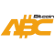Bitcoin ABC  0.22.13 P2P Digital Currency
schnorr_impl.h File Reference
`#include <string.h>`
`#include "schnorr.h"`
`#include "field.h"`
`#include "group.h"`
`#include "hash.h"`
`#include "ecmult.h"`
`#include "ecmult_gen.h"`
Include dependency graph for schnorr_impl.h:
This graph shows which files directly or indirectly include this file:

Go to the source code of this file.

## Functions

static int secp256k1_schnorr_sig_verify (const secp256k1_ecmult_context *ctx, const unsigned char *sig64, secp256k1_ge *pubkey, const unsigned char *msg32)
Custom Schnorr-based signature scheme. More...

static int secp256k1_schnorr_compute_e (secp256k1_scalar *e, const unsigned char *r, secp256k1_ge *p, const unsigned char *msg32)

static int secp256k1_schnorr_sig_sign (const secp256k1_context *ctx, unsigned char *sig64, const unsigned char *msg32, const secp256k1_scalar *privkey, secp256k1_ge *pubkey, secp256k1_nonce_function noncefp, const void *ndata)

static int secp256k1_schnorr_sig_generate_k (const secp256k1_context *ctx, secp256k1_scalar *k, const unsigned char *msg32, const secp256k1_scalar *privkey, secp256k1_nonce_function noncefp, const void *ndata)

## ◆ secp256k1_schnorr_compute_e()

 static int secp256k1_schnorr_compute_e ( secp256k1_scalar * e, const unsigned char * r, secp256k1_ge * p, const unsigned char * msg32 )
static

Definition at line 101 of file schnorr_impl.h.

Here is the call graph for this function:
Here is the caller graph for this function:

## ◆ secp256k1_schnorr_sig_generate_k()

 static int secp256k1_schnorr_sig_generate_k ( const secp256k1_context * ctx, secp256k1_scalar * k, const unsigned char * msg32, const secp256k1_scalar * privkey, secp256k1_nonce_function noncefp, const void * ndata )
static

Definition at line 176 of file schnorr_impl.h.

Here is the call graph for this function:
Here is the caller graph for this function:

## ◆ secp256k1_schnorr_sig_sign()

 static int secp256k1_schnorr_sig_sign ( const secp256k1_context * ctx, unsigned char * sig64, const unsigned char * msg32, const secp256k1_scalar * privkey, secp256k1_ge * pubkey, secp256k1_nonce_function noncefp, const void * ndata )
static

Negate the nonce if R.y is not a quadratic residue.

Definition at line 130 of file schnorr_impl.h.

Here is the call graph for this function:

## ◆ secp256k1_schnorr_sig_verify()

 static int secp256k1_schnorr_sig_verify ( const secp256k1_ecmult_context * ctx, const unsigned char * sig64, secp256k1_ge * pubkey, const unsigned char * msg32 )
static

Custom Schnorr-based signature scheme.

Signing: Inputs: 32-byte message m, 32-byte scalar key x (!=0) public key point P, 32-byte scalar nonce k (!=0)

Compute point R = k * G. Negate nonce if R.y is not a quadratic residue. Compute scalar e = Hash(R.x || compressed(P) || m) mod n. Compute scalar s = k + e * x. The signature is (R.x, s).

Verification: Inputs: 32-byte message m, public key point P, signature: (32-byte r, scalar s)

Signature is invalid if s >= n or r >= p. Compute scalar e = Hash(r || compressed(P) || m) mod n. Option 1 (faster for single verification): Compute point R = s * G - e * P. Reject if R is infinity or R.y is not a quadratic residue. Signature is valid if the serialization of R.x equals r. Option 2 (allows batch validation): Decompress x coordinate r into point R, with R.y a quadratic residue. Reject if R is not on the curve. Signature is valid if R + e * P - s * G == 0.

Definition at line 51 of file schnorr_impl.h.

Here is the call graph for this function: